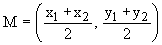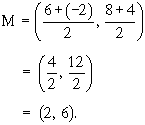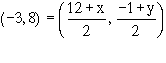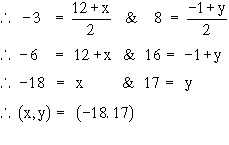Home MonkeyNotes Printable Notes Digital Library Study Guides Message Boards Study Smart Parents Tips College Planning Test Prep Fun Zone Help / FAQ How to Cite New Title Request

 9.5 Mid point Formula Theorem : If the co-ordinates of the end points of a segment are (x1, y1) and (x2, y2), then the co-ordinates of the mid point of this segment is given by the formula :Example 1 Find the mid point of a segment if A is (6,8) and B is (-2, 4). Solution : Let (6, 8) be (x1, y1) and (-2, 4) be (x2, y2). Therefore their mid point M is given as :Example 2 If the mid point of seg. AB is (-3, 8) and A is (12, -1), find co-ordinates of B. Solution : Let the co-ordinates of B be (x, y). According to the mid-point formulaTherefore the co-ordinates of B are (-18, 17). Index 9.1 Points And Co-ordinates 9. 2 Co-ordinates and Axes 9. 3 Quadrants 9. 4 Distances And Distances Formula 9. 5 Mid Point Formula 9. 6 Slope Of A Line 9. 7 Equation Of A Line Chapter 1
 Search: All Products Books Popular Music Classical Music Video DVD Toys & Games Electronics Software Tools & Hardware Outdoor Living Kitchen & Housewares Camera & Photo Cell Phones Keywords: